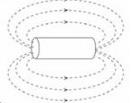Courses

# Test: Magnetic Field

## 10 Questions MCQ Test Physics For JEE | Test: Magnetic Field

Description
This mock test of Test: Magnetic Field for JEE helps you for every JEE entrance exam. This contains 10 Multiple Choice Questions for JEE Test: Magnetic Field (mcq) to study with solutions a complete question bank. The solved questions answers in this Test: Magnetic Field quiz give you a good mix of easy questions and tough questions. JEE students definitely take this Test: Magnetic Field exercise for a better result in the exam. You can find other Test: Magnetic Field extra questions, long questions & short questions for JEE on EduRev as well by searching above.
QUESTION: 1

### What is the angle of dip at a place where horizontal component of earth’s magnetic field is equal to the vertical component?

Solution:

The angle of dip is given by δ=tan−1(Bv​/Bh​)
where Bv​= vertical component of earth's magnetic field and
Bh= horizontal components of earth's magnetic field.
Here, Bv​=Bh
So, δ=tan−1(1)=45o

QUESTION: 2

### How can a magnetic field be produced?

Solution:

An electric current as well as the permanent magnet produces a magnetic field whereas a temporary magnet fails to do so.

QUESTION: 3

### Which one of the Maxwell’s laws leads to the conclusion that there are no magnetic field loops that are not closed?

Solution:

Gauss’s law for magnetism states that no magnetic monopoles exists and that the total flux through a closed surface must be zero.

QUESTION: 4

What is the relation between dipole moment pm, volume of bar magnet V and intensity of magnetization M?

Solution:

Magnetization is the net dipole moment aligned per unit volume.

Hence B

QUESTION: 5

If we place a bar magnet in the magnetic meridian with its north pole towards geographic north, the neutral point will be:

Solution:

If we place a bar magnet in the magnetic meridian with its north pole towards geographic north, the neutral point will be on the equatorial line of the magnet. If the north pole of the magnet points towards geographical north, the fields of the magnet and the earth will point in opposite directions along the equatorial line of the magnet we get two neutral points on the equatorial line which are equidistant from the axis of the magnet.

QUESTION: 6

Which combination of magnetic field lines and poles shows two magnets repelling each other?

Solution:

The discovery that one particular pole of a magnet orients northward, whereas the other pole orients southward allowed people to identify the north and south poles of any magnet. It was then noticed that the north poles of two different magnets repel each other, and likewise for the south poles. Conversely, the north pole of one magnet attracts the south pole of other magnets. This situation is analogous to that of electric charge, where like charges repel and unlike charges attract. In magnets, we simply replace charge with pole: Like poles repel and unlike poles attract.

QUESTION: 7

Magnetic field strength due to a short bar magnet on its axial line at a distance x is B. What is its value at the same distance on the equatorial line?

Solution:

A is the correct option.
The magnetic field at any axial point is given by B =  2μo/ 4πx3

Similarly, field at any equatorial point is given by, B = μo/ 4πx3

Thus, field at any equatorial point is half of what it is at an axial point.

QUESTION: 8

When the switch is closed a magnetic field is produced by the coil. Which answer shows the shape of the field?

Solution:

B is the correct option.The apparatus used by Faraday to demonstrate that magnetic fields can create currents is illustrated in Figure. When the switch is closed, a magnetic field is produced in the coil on the top part of the iron ring and transmitted to the coil on the bottom part of the ring.QUESTION: 9

If the magnet is suspended at an angle 30° to the magnetic meridian, the dip needle makes an angle 60o with the horizontal. What is the true dip?​

Solution:

Answer :- b

Solution :- Angle of dip, δ=30o

∴ tanδ′ = tanδ/cosθ

tanδ = tan 60*cos30o = [(3)^½]*[(3)^1/2/2]​

= 3/2

∴ Real dip δ′ = tan−1 (3/2)

QUESTION: 10

A compass has a small balanced pointer that always points North-South. This is because:

Solution:

The correct answer is Option B.
A compass a small magnet inside it with its north pole towards the tip of the needle.
This magnet aligns itself with earth's magnetic field thus points towards magnetic south and geographical north.